NEET  >  31 Year NEET Previous Year Questions: Waves - 2

# 31 Year NEET Previous Year Questions: Waves - 2

Test Description

## 30 Questions MCQ Test Physics Class 11 | 31 Year NEET Previous Year Questions: Waves - 2

31 Year NEET Previous Year Questions: Waves - 2 for NEET 2023 is part of Physics Class 11 preparation. The 31 Year NEET Previous Year Questions: Waves - 2 questions and answers have been prepared according to the NEET exam syllabus.The 31 Year NEET Previous Year Questions: Waves - 2 MCQs are made for NEET 2023 Exam. Find important definitions, questions, notes, meanings, examples, exercises, MCQs and online tests for 31 Year NEET Previous Year Questions: Waves - 2 below.
Solutions of 31 Year NEET Previous Year Questions: Waves - 2 questions in English are available as part of our Physics Class 11 for NEET & 31 Year NEET Previous Year Questions: Waves - 2 solutions in Hindi for Physics Class 11 course. Download more important topics, notes, lectures and mock test series for NEET Exam by signing up for free. Attempt 31 Year NEET Previous Year Questions: Waves - 2 | 30 questions in 60 minutes | Mock test for NEET preparation | Free important questions MCQ to study Physics Class 11 for NEET Exam | Download free PDF with solutions
 1 Crore+ students have signed up on EduRev. Have you?
31 Year NEET Previous Year Questions: Waves - 2 - Question 1

### A cylindrical resonance tube open at both ends, has a fundamental frequency, f, in air. If half of the length is dipped vertically in water, the fundamental frequency of the air column will be

Detailed Solution for 31 Year NEET Previous Year Questions: Waves - 2 - Question 1

Fundamental frequency of open pipe,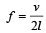When half of tube is filled with water, then the length of air column becomes half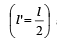and the pipe becomes closed.

So,  new fundamental frequency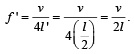Clearly f ' = f.

31 Year NEET Previous Year Questions: Waves - 2 - Question 2

### A transverse wave is represented by the equation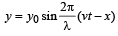For what value of λ is the maximum particle velocity equal to two times the wave velocity? 

Detailed Solution for 31 Year NEET Previous Year Questions: Waves - 2 - Question 2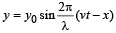Particle velocity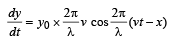Maximum particle velocity =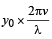Wave velocity = v [given]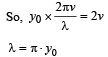31 Year NEET Previous Year Questions: Waves - 2 - Question 3

### A vehicle, with a horn of frequency n is moving with a velocity of 30 m/s in a direction perpendicular to the straight line joining the observer and the vehicle. The observer perceives the sound to have a frequency n + n1.Then (if the sound velocity in air is 300 m/s) 

Detailed Solution for 31 Year NEET Previous Year Questions: Waves - 2 - Question 3

As the source is not moving towards or away from the observer in a straight line, so the Doppler’s effect will not be observed by the observer.

31 Year NEET Previous Year Questions: Waves - 2 - Question 4

A standing wave having 3 nodes and 2 antinodes is formed between two atoms having a distance 1.21 Å between them. The wavelength of the standing wave is 

Detailed Solution for 31 Year NEET Previous Year Questions: Waves - 2 - Question 4

Let  be length of string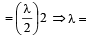Hence, the wave length of standing wave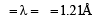31 Year NEET Previous Year Questions: Waves - 2 - Question 5

In a sinusoidal wave, the time required for a particular point to move from maximum displacement to zero displacement is 0.170 sec.The frequency of the wave is 

Detailed Solution for 31 Year NEET Previous Year Questions: Waves - 2 - Question 5

Time taken to move from maximum to zero displacement =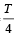∴ Time period T = 4 × 0.170 second

∴  Frequency,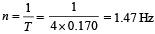31 Year NEET Previous Year Questions: Waves - 2 - Question 6

Resonance is an example of 

Detailed Solution for 31 Year NEET Previous Year Questions: Waves - 2 - Question 6

We know that if frequency of an external forced  oscillation is equal to the natural frequency of the body, then amplitude of the forced oscillation of the body becomes very large. This phenomenon is known as resonant vibration. Therefore, resonance is an example of forced vibration.

31 Year NEET Previous Year Questions: Waves - 2 - Question 7

Two waves of lengths 50 cm and 51 cm produce 12 beats per sec. The velocity of sound is  

Detailed Solution for 31 Year NEET Previous Year Questions: Waves - 2 - Question 7

Given : Wavelength of first wave (λ1) = 50 cm = 0.5 m Wavelength of second wave (λ2) = 51 cm = 0.51m

frequency of beats per sec (n) = 12.
We know that the frequency of beats,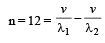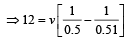= v[2 - 1.9608]  = v x 0.0392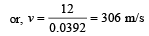[where, v = velocity of sound]

31 Year NEET Previous Year Questions: Waves - 2 - Question 8

If n1, n2 and n3 are the fundamental frequencies of three segments into which a string is divided, then the original fundamental frequency n of the string is given by 

Detailed Solution for 31 Year NEET Previous Year Questions: Waves - 2 - Question 8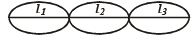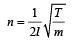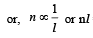= constant, K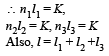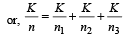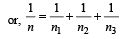31 Year NEET Previous Year Questions: Waves - 2 - Question 9

Two sound sources emitting sound each of wavelength λ are fixed at a given distance apart.
A listener moves with a velocity u along the line joining the two sources. The number of beats heard by him per second is 

Detailed Solution for 31 Year NEET Previous Year Questions: Waves - 2 - Question 9

Frequency received by listener from the rear source,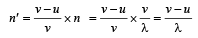Frequency received by listener from the front source,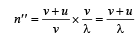No. of beats = n'' – n'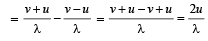31 Year NEET Previous Year Questions: Waves - 2 - Question 10

The equation of a wave is represented by: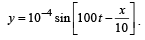The velocity of the wave will be 

Detailed Solution for 31 Year NEET Previous Year Questions: Waves - 2 - Question 10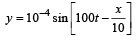v = 100 sec–1, λ = 10 m

v = λv = 10 × 100 = 1000 m/s

31 Year NEET Previous Year Questions: Waves - 2 - Question 11

A source and an observer move away from each other, with a velocity of 10m/s with respect to ground. If the observer finds the frequency of sound coming from the source as 1950 Hz, then original frequency of source is (velocity of sound in air = 340 m/s) 

Detailed Solution for 31 Year NEET Previous Year Questions: Waves - 2 - Question 11

According to Doppler's effect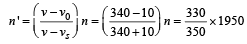= 2068 Hz

31 Year NEET Previous Year Questions: Waves - 2 - Question 12

A string of 7 m length has a mass of 0.035 kg. If tension in the string is 60.5 N, then speed of a wave on the string is 

Detailed Solution for 31 Year NEET Previous Year Questions: Waves - 2 - Question 12

Given : Length (l) = 7 m
Mass (M) = 0.035 kg and tension (T) = 60.5 N.
Therefore, mass of string per unit length (m)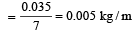speed of wave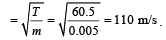31 Year NEET Previous Year Questions: Waves - 2 - Question 13

The equation for a transverse wave travelling along the positive x-axis with amplitude 0.2 m, velocity v =360 ms–1 and wavelength λ = 60 m can be written as 

Detailed Solution for 31 Year NEET Previous Year Questions: Waves - 2 - Question 13

v = nλ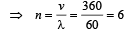a = 0.2

For a wave travelling along positive x-axis y  = a sin (ωt – kx)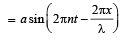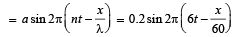31 Year NEET Previous Year Questions: Waves - 2 - Question 14

A whistle of frequen cy 385 Hz rotates in a horizontal circle of radius 50 cm at an angular speed of 20 radians s–1. The lowest frequency heard by a listener a long distance away at rest with respect to the centre of the circle, given velocity of sound equal to 340 ms–1, is 

Detailed Solution for 31 Year NEET Previous Year Questions: Waves - 2 - Question 14

Velocity of source

vs = rω =  0.50 x 20 = 10 ms-1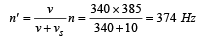31 Year NEET Previous Year Questions: Waves - 2 - Question 15

An observer moves towards a stationary source of sound with a speed 1/5th of the speed of sound. The wavelength and frequency of the sound emitted are λ and f respectively. The apparent frequency and wavelength recorded by the observer are respectively. 

Detailed Solution for 31 Year NEET Previous Year Questions: Waves - 2 - Question 15Wavelength remains constant (unchanged) in this case

31 Year NEET Previous Year Questions: Waves - 2 - Question 16

A car is moving towards a high cliff. The car driver sounds a horn of frequency f. The reflected sound heard by the driver has frequency 2f. If v be the velocity of sound, then the velocity of the car, in the same velocity units, will be

Detailed Solution for 31 Year NEET Previous Year Questions: Waves - 2 - Question 16

Let f ' be the frequency of sound heard by cliff.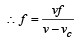....(1)

Now, for the reflected wave, cliff acts as a source,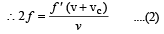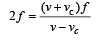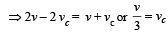31 Year NEET Previous Year Questions: Waves - 2 - Question 17

The phase difference between two waves, represented by 
y1 = 10–6 sin{100 t + (x/50) + 0.5} m
y2 = 10–6 cos{100 t + (x/50)} m
where x is expressed in metres and t is expressed in seconds, is approximately 

Detailed Solution for 31 Year NEET Previous Year Questions: Waves - 2 - Question 17

y1 = 10–6 sin (100t + x/50 + 0.5)m
= 10–6 cos (100t + x/50 – π/2 + 0.5)m
y2  = 10–6 cos (100t + x/50)m

∴ φ = π/2 – 0.5 = 1.07 rad

31 Year NEET Previous Year Questions: Waves - 2 - Question 18

Two vibrating tuning for ks produce progressive waves given by y1 = 4 sin 500 πt and y2 = 2 sin 506 πt. Number of beats produced per minute is [2 00 5]

Detailed Solution for 31 Year NEET Previous Year Questions: Waves - 2 - Question 18

Equation of progressive wave is given by y = A sin2πft
Given y1 = 4sin500 πt and y2 = 2sin506πt.
Comparing the given equations with equation of progressive wave, we get 2f1 = 500 ⇒  f1 = 250 2f2 = 506 ⇒ f2 = 253
Beats = f2– f1 = 253 – 250 = 3 beats/sec = 3 × 60 = 180 beats/minute.

31 Year NEET Previous Year Questions: Waves - 2 - Question 19

A point source emits sound equally in all directions in a non–absorbing medium. Two points P and Q are at distances of 2m and 3m respectively from the source. The ratio of the intensities of the waves at P and Q is 

Detailed Solution for 31 Year NEET Previous Year Questions: Waves - 2 - Question 19

Intensity = Energy/sec/unit area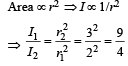31 Year NEET Previous Year Questions: Waves - 2 - Question 20

Which one of the following statements is true ? 

Detailed Solution for 31 Year NEET Previous Year Questions: Waves - 2 - Question 20

Sound waves in air are longitudinal and the light waves are transverse.

31 Year NEET Previous Year Questions: Waves - 2 - Question 21

A transverse wave propagating along x-axis is represented by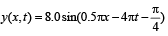where x is in metres and t is in seconds. The speed of the wave is 

Detailed Solution for 31 Year NEET Previous Year Questions: Waves - 2 - Question 21

Speed of a wave represented by the equation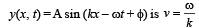By comparison, ω = 4π; k = 0.5π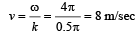31 Year NEET Previous Year Questions: Waves - 2 - Question 22

The time of reverberation of a room A is one second. What will be the time (in seconds) of reverberation of a room, having all the dimensions double of those of room A ?

Detailed Solution for 31 Year NEET Previous Year Questions: Waves - 2 - Question 22

Reverberation time is defined as the time during which the intensity of sound in the auditorium becomes one millionth of the initial intensity.
Sabine has shown that standard reverberation time for an auditorium is given by the formula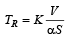Here, V is volume of the auditorium, S is the surface area. So,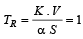(given)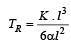(Assuming auditorium to be cubic in shape)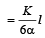So, TR α l

If dimension is doubled, reverberation time t will be doubled. So, New TR = 2 sec.

31 Year NEET Previous Year Questions: Waves - 2 - Question 23

Two sound waves with wavelengths 5.0 m and 5.5m respectively, each propagate in a gas with velocity 330 m/s. We expect the following number of beats per second 

Detailed Solution for 31 Year NEET Previous Year Questions: Waves - 2 - Question 23

Frequencies of sound waves are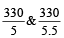i.e.,  66 Hz and 60 Hz
Frequencies of beat = 66 – 60 = 6 per second

31 Year NEET Previous Year Questions: Waves - 2 - Question 24

Two points are located at a distance of 10 m and 15 m from the source of oscillation. The period of oscillation is 0.05 sec and the velocity of the wave is 300 m/sec. What is the phase difference between the oscillations of two points? 

Detailed Solution for 31 Year NEET Previous Year Questions: Waves - 2 - Question 24

Here, T = 0.05 sec, v = 300 ms–1.
Now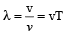= (300 x 0.05)m

or, λ = 15 m

Phase of the point at 10 m from the source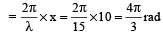Phase of the point at 15 m from the source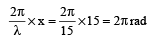∴ The phase difference between the points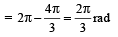31 Year NEET Previous Year Questions: Waves - 2 - Question 25

The wave described by y = 0.25 sin (10πx – 2πt), where x and y are in meters and t in seconds, is a wave travelling along the: 

Detailed Solution for 31 Year NEET Previous Year Questions: Waves - 2 - Question 25

y = 0.25 sin (10πx – 2πt)

Comparing this equation with the standard wave equation y = asin (kx – ωt)

We get,  k = 10π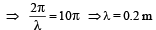And ω = 2π or, 2πv = 2π ⇒ v = 1Hz.
The sign inside the bracket is negative, hence the wave travels in + ve x- direction.

31 Year NEET Previous Year Questions: Waves - 2 - Question 26

Each of the two strings of length 51.6 cm and 49.1 cm are tensioned separately by 20 N force.Mass per unit length of both the strings is same and equal to 1 g/m. When both the strings vibrate simultaneously the number of beats is

Detailed Solution for 31 Year NEET Previous Year Questions: Waves - 2 - Question 26

The frequency of vibration of a string is
given by,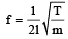where m is mass per unit length.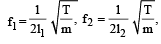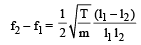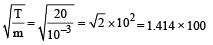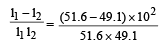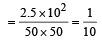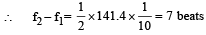31 Year NEET Previous Year Questions: Waves - 2 - Question 27

The driver of a car travelling with speed 30 m/sec towards a hill sounds a horn of frequency 600 Hz. If the velocity of sound in air is 330 m/s, the frequency of reflected sound as heard by driver is 

Detailed Solution for 31 Year NEET Previous Year Questions: Waves - 2 - Question 27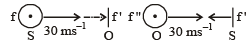f' is the apparent frequency received by an observer at the hill. f'' is the frequency of the reflected sound as heard by driver.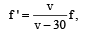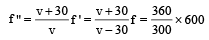= 720 Hz

31 Year NEET Previous Year Questions: Waves - 2 - Question 28

A wave in a string has an amplitude of 2 cm. The wave travels in the + ve direction of  x axis with a speed of 128 m/sec and it is noted that 5 complete waves fit in 4 m length of the string.The equation describing the wave is 

Detailed Solution for 31 Year NEET Previous Year Questions: Waves - 2 - Question 28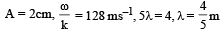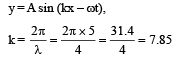y = 0.02 m sin (7.857 – 1005 t)

ω  = 128 × 7.85 = 1005

31 Year NEET Previous Year Questions: Waves - 2 - Question 29

A transverse wave is represented by y = A sin (ωt–kx). For what value of the wavelength is the wave velocity equal to the maximum particle velocity? 

Detailed Solution for 31 Year NEET Previous Year Questions: Waves - 2 - Question 29

y = A sin (ωt–kx)

Particle velocity,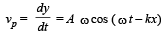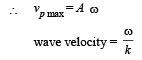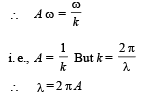31 Year NEET Previous Year Questions: Waves - 2 - Question 30

A tuning fork of freqqency 512 Hz makes 4 beats per second with the vibrating string of a piano.The beat frequency decreases to 2 beats per sec when the tension in the piano string is slightly increased. The frequency of the piano string before increasing the tension was 

Detailed Solution for 31 Year NEET Previous Year Questions: Waves - 2 - Question 30

The frequency of the pianostring = 512 ± 4 = 516 or 508. When the tension is increased, beat frequency decreases to 2, it means that frequency of the string is 508 as frequency of string increases with tension.

## Physics Class 11

127 videos|464 docs|210 tests
 Use Code STAYHOME200 and get INR 200 additional OFF Use Coupon Code
Information about 31 Year NEET Previous Year Questions: Waves - 2 Page
In this test you can find the Exam questions for 31 Year NEET Previous Year Questions: Waves - 2 solved & explained in the simplest way possible. Besides giving Questions and answers for 31 Year NEET Previous Year Questions: Waves - 2, EduRev gives you an ample number of Online tests for practice

## Physics Class 11

127 videos|464 docs|210 tests

### How to Prepare for NEET

Read our guide to prepare for NEET which is created by Toppers & the best Teachers